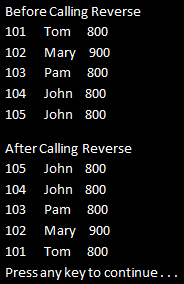Support us .Net Basics C# SQL ASP.NET Aarvi MVC Slides C# Programs Subscribe Download

### Part 11 - Ordering Operators in LINQ - II

Suggested Videos
Part 8 - SelectMany Operator
Part 9 - Difference between Select and SelectMany
Part 10 - Ordering Operators in LINQ

This is continuation to Part 10. Please watch Part 10 before proceeding.

The following 5 standard LINQ query operators belong to Ordering Operators category
OrderBy
OrderByDescending
ThenBy
ThenByDescending
Reverse

In Part 10, we discussed OrderBy & OrderByDescending operators. In this video we will discuss
ThenBy
ThenByDescending
Reverse

OrderBy, OrderByDescending, ThenBy, and ThenByDescending can be used to sort data. Reverse method simply reverses the items in a given collection.

We will use the following Student class in this demo.
public class Student
{
public int StudentID { get; set; }
public string Name { get; set; }
public int TotalMarks { get; set; }

public static List<Student> GetAllStudetns()
{
List<Student> listStudents = new List<Student>
{
new Student
{
StudentID= 101,
Name = "Tom",
TotalMarks = 800
},
new Student
{
StudentID= 102,
Name = "Mary",
TotalMarks = 900
},
new Student
{
StudentID= 103,
Name = "Pam",
TotalMarks = 800
},
new Student
{
StudentID= 104,
Name = "John",
TotalMarks = 800
},
new Student
{
StudentID= 105,
Name = "John",
TotalMarks = 800
},
};

return listStudents;
}
}

OrderBy or OrderByDescending work fine when we want to sort a collection just by one value or expression.

If want to sort by more than one value or expression, that's when we use ThenBy or ThenByDescending along with OrderBy or OrderByDescending.

OrderBy or OrderByDescending performs the primary sort. ThenBy or ThenByDescending is used for adding secondary sort. Secondary Sort operators (ThenBy or ThenByDescending ) can be used more than once in the same LINQ query.

Example 1:
a) Sorts Students first by TotalMarks in ascending order(Primary Sort)
b) The 4 Students with TotalMarks of 800, will then be sorted by Name in ascending order (First Secondary Sort)
c) The 2 Students with Name of John, will then be sorted by StudentID in ascending order (Second Secondary Sort)

IEnumerable<Student> result = Student.GetAllStudetns()
.OrderBy(s => s.TotalMarks).ThenBy(s => s.Name).ThenBy(s => s.StudentID);
foreach (Student student in result)
{
Console.WriteLine(student.TotalMarks + "\t" + student.Name + "\t" + student.StudentID);
}

Output:Example 2: Rewrite Example 1 using SQL like syntax. With SQL like syntax we donot use ThenBy or ThenByDescending, instead we specify the sort expressions using a comma separated list. The first sort expression will be used for primary sort and the subsequent sort expressions for secondary sort.

IEnumerable<Student> result = from student in Student.GetAllStudetns()
orderby student.TotalMarks, student.Name, student.StudentID
select student;
foreach (Student student in result)
{
Console.WriteLine(student.TotalMarks + "\t" + student.Name + "\t" + student.StudentID);
}

Example 3: Reverses the items in the collection.

IEnumerable<Student> students = Student.GetAllStudetns();

Console.WriteLine("Before calling Reverse");
foreach (Student s in students)
{
Console.WriteLine(s.StudentID + "\t" + s.Name + "\t" + s.TotalMarks);
}

Console.WriteLine();
IEnumerable<Student> result = students.Reverse();

Console.WriteLine("After calling Reverse");
foreach (Student s in result)
{
Console.WriteLine(s.StudentID + "\t" + s.Name + "\t" + s.TotalMarks);
}

Output:1.Nice Tutorial.
all videos are very helpful and best for the freshers.

2.b) The 4 Students with TotalMarks of 800,

This condition was missed

3.when we can short by multiple fields by order by (comma seperated)
then why need to use THENBY. means orderby able to do work of thenby .Please Clarify....
IEnumerable result = from student in Student.GetAllStudetns()
orderby student.TotalMarks, student.Name, student.StudentID

4.Hi manoj , the comma seperated list works with sql query syntax,, but not with LINQ,,
And ThenBy works with LINQ but not with Sql query syntax..
so check it once..

It would be great if you can help share these free resources